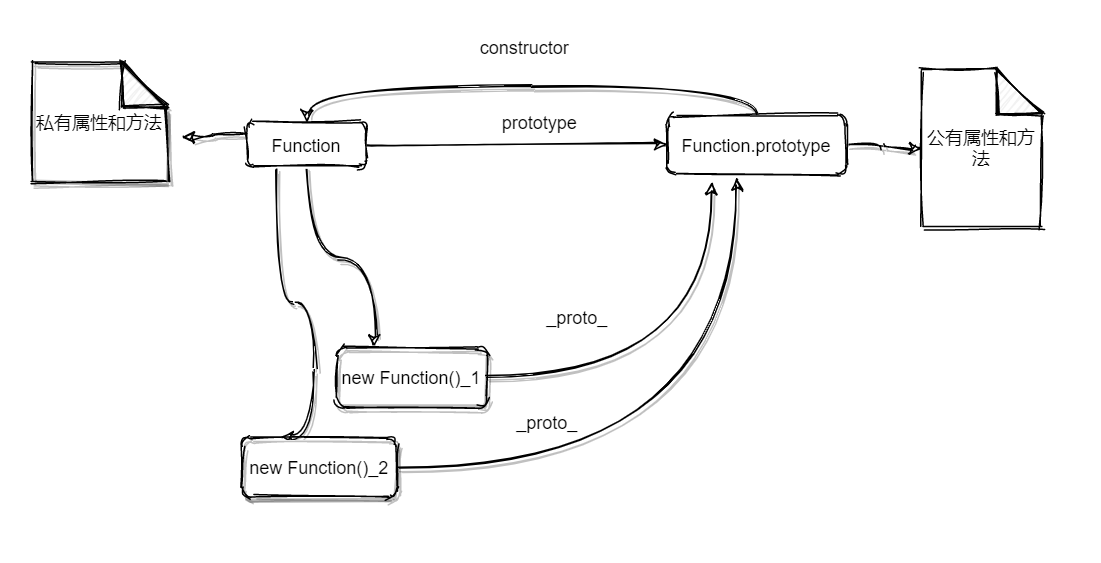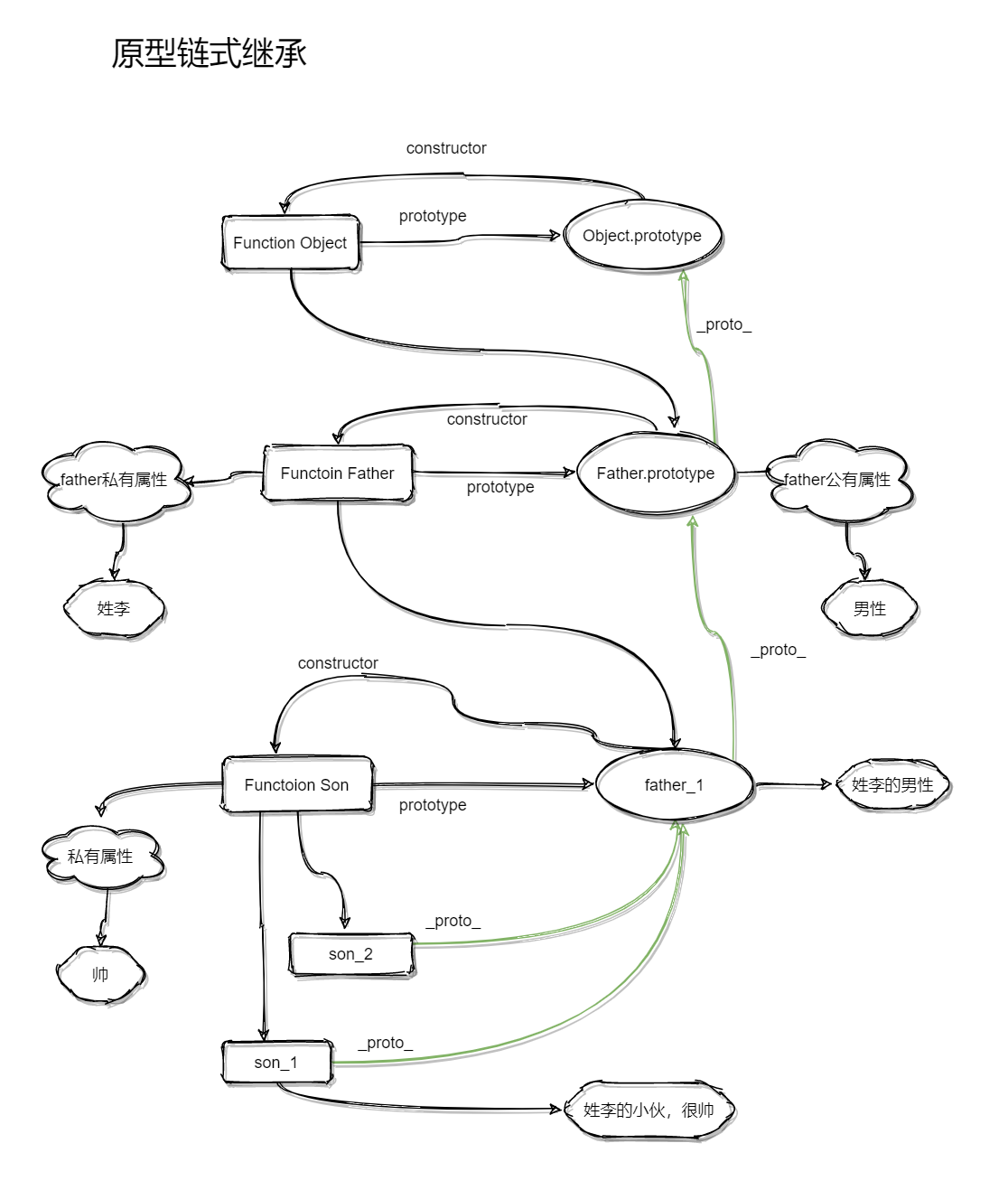# JavaScript面向对象程序设计之继承（一）

## 1. 原型链式继承

### 1.1 原型模式

function Person (name) {
this.name = name // 私有属性
}
// 公共方法
Person.prototype.sayName = function () {
console.log(this.name)
}

// 创建实例
var personA = new Person('A')
console.log(personA.name) // A
personA.sayName() // A

var personB = new Person('B')
console.log(personB.name) // B
personB.sayName() // B### 1.2.1 原型链与原型链式继承

function Person (name) {
this.name = name
}
Person.prototype = {
constructor: Person,
sayName: function () {
console.log(this.name + '-hello')
}
}
var personA = new Person('A')
personA.sayName() // A-hellofunction SuperType () {
this.superName = 'superName'
}
SuperType.prototype.sayName = function () {
console.log(this.name)
}
function SubType (subName) {
this.name = subName || 'subName'
}
SubType.prototype = new SuperType()
var subA = new SubType('A')
subA.sayName() // A
console.log(subA.superName) // superName


### 1.2.2 原型链式继承的缺陷

function SuperType () {
this.element = ['A', 'B', 'C']
}
SuperType.prototype.printElement = function () {
console.log(this.element)
}
function SubType () {
}
SubType.prototype = new SuperType()

var subA = new SubType()
subA.element.push('D')
subA.printElement() // ["A", "B", "C", "D"]
var subB = new SubType()
subB.element.push('E')
subB.printElement() // ["A,", "B", "C", "D","E"]


## 2. 借用构造函数

### 2.1 借用构造函数实现继承

function SuperType (name) {
this.name = name || 'superName'
this.element = ['A', 'B', 'C']
}
function SubType (name) {
SuperType.apply(this, arguments) // SuperType.call(this, name)
}

var subA = new SubType('subA')
subA.element.push('D')
console.log(subA.element) // ["A", "B", "C", "D"]
console.log(subA.name) // subA
var subB = new SubType('subB')
subB.element.push('E')
console.log(subB.element) // ["A", "B", "C", "E"]
console.log(subB.name) // subB


function SubType () {
this.propertyA = 'propertyA'
this.propertyB = 'propertyB'
this.propertyC = 'propertyC'
this.propertyD = 'propertyD'
this.propertyE = 'propertyE'
}


function SubType () {
SuperType()
}
function SuperType () {
this.propertyA = 'propertyA'
this.propertyB = 'propertyB'
this.propertyC = 'propertyC'
this.propertyD = 'propertyD'
this.propertyE = 'propertyE'
}


SubType构造函数内部调用SuperType构造函数，将SuperType中的属性复用到SubType中。

function SubType () {
window.propertyA = 'propertyA'
window.propertyB = 'propertyB'
window.propertyC = 'propertyC'
window.propertyD = 'propertyD'
window.propertyE = 'propertyE'
}


function SubType () {
SuperType()
}
function SuperType () {
this.propertyA = 'propertyA'
this.propertyB = 'propertyB'
this.propertyC = 'propertyC'
this.propertyD = 'propertyD'
this.propertyE = 'propertyE'
}

var subA = new SubType()
console.log(subA.propertyA) // undefined
console.log(window.propertyA) // propertyA


function SubType () {
SuperType.apply(this,arguments)
}
function SuperType () {
this.propertyA = 'propertyA'
this.propertyB = 'propertyB'
this.propertyC = 'propertyC'
this.propertyD = 'propertyD'
this.propertyE = 'propertyE'
}

var subA = new SubType()
console.log(subA.propertyA) // propertyA
console.log(window.propertyA) // propertyA


• 每个实例都可以保持超类型中自有属性的私有性，每个子类实例中都可以保有超类型中自有属性的一个副本，子类实例之间对继承而来的自有属性的操作不会相互干扰；
• 子类型的构造函数可以向超类型的构造函数中传递参数；

### 2.2 借用构造函数的缺陷

function SuperType (name) {
this.name = name || 'superName'
this.element = ['A', 'B', 'C']
}
SuperType.prototype.sayName = function () {
console.log(this.name)
}
function SubType (name) {
SuperType.apply(this, arguments) // SuperType.call(this, name)
}

var subA = new SubType('subA')
subA.sayName() // Uncaught TypeError: subA.sayName is not a function


function SuperType (name) {
this.name = name || 'superName'
this.element = ['A', 'B', 'C']
this.sayName = function () {
console.log(this.name)
}
}
function SubType (name) {
SuperType.apply(this, arguments) // SuperType.call(this, name)
}
var subA = new SubType('subA')
subA.sayName() // 'subA'
var subB = new SubType('subB')
subB.sayName() // 'subB'


console.log(subA.sayName === subB.sayName) // false


• 无法定义子类型可复用的公共方法；
• 无法访问超类型的原型；

## 3. 组合继承

### 3.1 组合继承

function SuperType (name) {
this.name = name || 'superName'
this.element = ['A', 'B', 'C']
}
SuperType.prototype.sayName = function () {
console.log(this.name)
}
SuperType.prototype.printElement = function () {
console.log(this.element)
}
function SubType (name) {
SuperType.apply(this, arguments)
}
SubType.prototype = new SuperType ()
var subA = new SubType('subA')
subA.element.push('subA')
subA.sayName() // subA
subA.printElement() // ["A", "B", "C", "subA"]
var subB = new SubType('subB')
subB.element.push('subB')
subB.sayName() // subB
subB.printElement() // ["A", "B", "C", "subB"]
console.log(subA.sayName === subB.sayName) // true


### 3.2 组合继承的缺陷

posted @ 2021-01-28 13:29  CherishTheYouth  阅读(126)  评论(1编辑  收藏  举报